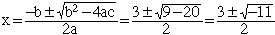Name: Katy Who is asking: Student Level: Secondary Question: How do you find the solution to x2-3x+5=0? Hi Katy, You can try completing the square or using the general quadratic formula. To complete the square add 9/4 to both sides to get x2 - 3x + 9/4 + 5 = 9/4 which is (x - 3/2)2 + 5 = 9/4 or (x - 3/2)2 = - 5 + 9/4 = -11/4 Here you see the problem, (x - 3/2)2 cannot be negative. If you try to solve the equation using the general quadratic you getand you have the same problem, finding the square root of a negative number. Thus you have two choices. If you are restricting yourself to real numbers then the answer is that there is no solution. If you allow complex numbers, that is you allow for taking the square roots of negative numbers, then there is a pair of solutions given in the general quadtatic expression above. I hope this helps, Penny Go to Math Central